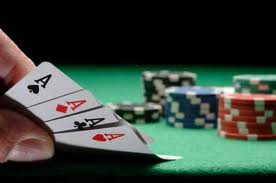# Hutchinson Point Count System for Omaha High/Low

In 1997 Edward Hutchinson published a formula in Canadian Poker Monthly for determining which starting hands in Omaha high/low are significantly more likely to win than random hands. I used it extensively when I was learning to play and think it is worth a read for all new players.

It is based on a model assuming a ten handed loose low level game of limit omaha high/low with lots of people standing to the end of each hand. As always in poker there is no invincible strategy for all situations. Use it as a guide not a rulebook.

#### Omaha High/Low

A high hand can only win the high half of a pot (scooping if no low qualifies). The best low hand may win both halves of the pot because you are allowed to use different combinations of cards to determine your best high and low hands.#### High-Only Hands

The first step is to decide if a hand qualifies as a high-only hand. A hand is a playable high-only hand if it meets all of the following conditions:

1. All four cards are tens or better ( A K Q J T)
2. It contains at least one of the following
• two pairs
• a pair and two cards of the same suit
• two cards of one suit and two cards of another suit
3. It does not contain a set (three cards of the same rank) or quads

#### Two-Way Hands

If a hand fails to qualify as a high-only hand, the next step is to decide if it may be played as a two-way hand. To do this you award the four hole cards points using the following scale:

1. For the two smallest cards of different ranks
• Ace Two = 20 points
• Ace Three = 17 points
• Ace Four = 13 points
• Ace Five = 10 points
• Two Three = 15 points
• Two Four = 12 points
• Three Four = 11 points
• Four Five = 8 points
• All Others = 0 points
2. For the two remaining “side cards” using each rank only once
• A Three = 9 points
• A Four = 6 points
• A Five = 4 points
• A Jack, Queen or King = 2 points
• A Six or Ten = 4 points
• A Seven, Eight or Nine = 0 points
3. For any pair in the hand
• Aces = 8 points
• Kings = 6 points
• Queens = 5 points
• Jacks = 2 points
• Tens = 1 point
• Fours or Threes = 1 point
• Twos = 3 points
• All Others = 0 points
• If the hand contains a set (3 cards of the same rank) deduct half the points from this step
• If the hand contains quads deduct all the points from this step
4. If the hand any two suited cards
• Ace High = 4 points
• King High = 3 points
• Queen or Jack High = 2 points
• Ten, Nine or Eight High = 1 point
• All Others = 0 points
• If the hand contains three cards of the same suit deduct half the points from this step
• If the hand contains four cards of the same suit deduct all the points from this step

#### Hutchinson Point Count Scoring System

The maxmium possible score is 45 points. Edward Hutchinson recommends you only play hands which score twenty points or more. You can consider raising preflop if you are dealt a combination of cards which score thirty points or more

Next we look at some sample hands. We also examine the Premium Starting Hands in Omaha High/Low and how they score under the Hutchinson Point Count system.